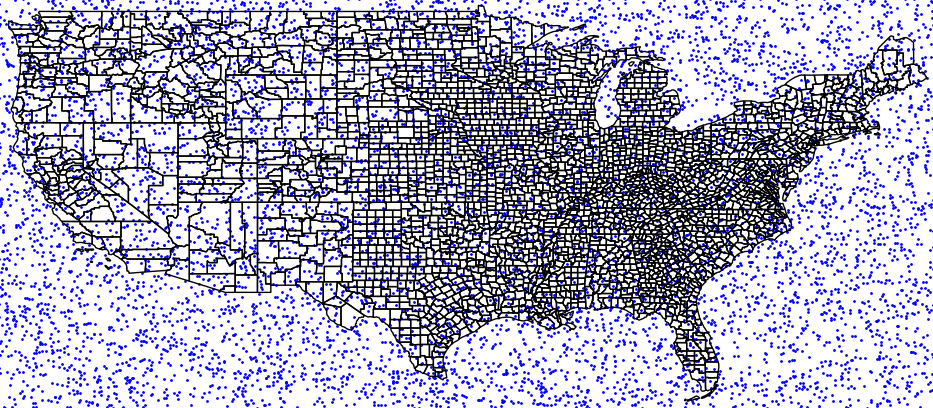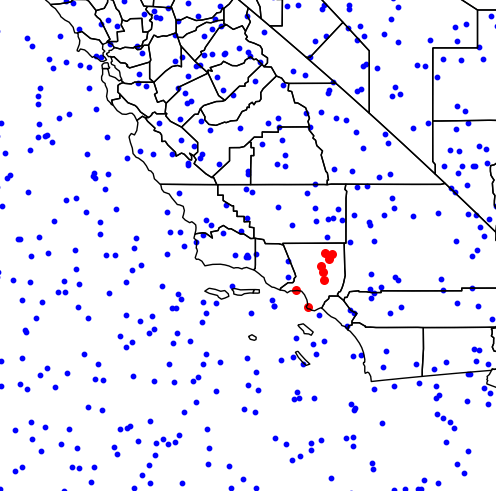# How to Run Fast Point-in-Polygon Tests with Python

A tutorial for performing fast point-in-polygon tests with Python's Shapeley and Geopandas.Performing point-in-polygon tests with Python with shapeley, geopandas, pygeos and Rtree

Performing point-in-polygon queries is one of the most commonplace operations in geospatial analysis and GIS. As Python is a great tool for automating GIS workflows, being able to solve this basic problem of computational geometry as efficiently as possible is a prerequisite for further analysis.

## How to check if a point is inside a polygon in Python

To perform a Point in Polygon (PIP) query in Python, we can resort to the Shapely library’s functions .within(), to check if a point is within a polygon, or .contains(), to check if a polygon contains a point.

Here’s some example code on how to use Shapely.

from shapely.geometry import Point, Polygon

# Create Point objects
p1 = Point(24.82, 60.24)
p2 = Point(24.895, 60.05)

# Create a square
coords = [(24.89, 60.06), (24.75, 60.06), (24.75, 60.30), (24.89, 60.30)]
poly = Polygon(coords)

# PIP test with 'within'
p1.within(poly)     # True
p2.within(poly)     # False

# PIP test with 'contains'
poly.contains(p1)   # True
poly.contains(p2)   # False 

## How to get a list of points inside a polygon in Python

One of the simplest and most efficient ways of getting a list of points inside a polygon with Python is to rely on the Geopandas library and perform a ‘spatial join’. Simply create one geodataframe with points and another one with polygons, and join them with ‘sjoin’.

In this example, we will use, as polygons, the shapefile of US counties and a list of random points, uniformly distributed in the lat-long region of (23,51)x(-126,-64).

import geopandas as gpd
import pandas as pd
import numpy as np
import matplotlib.pyplot as plt
from shapely.geometry import Point

# Open the shapefile
counties = gpd.GeoDataFrame.from_file('cb_2018_us_county_20m.shp')

# Generate random points
N=10000
lat = np.random.uniform(23,51,N)
lon = np.random.uniform(-126,-64,N)

# Create geodataframe from numpy arrays
df = pd.DataFrame({'lon':lon, 'lat':lat})
df['coords'] = list(zip(df['lon'],df['lat']))
df['coords'] = df['coords'].apply(Point)
points = gpd.GeoDataFrame(df, geometry='coords', crs=counties.crs)

# Perform spatial join to match points and polygons
pointInPolys = gpd.tools.sjoin(points, counties, op="within", how='left')

That’s it, we now have matched points and polygons. For example use in how to retrieve the points from a specific polygon, we can continue as follows:

# Example use: get points in Los Angeles, CA.
pnt_LA = points[pointInPolys.NAME=='Los Angeles']

# Plot map with points in LA in red
base = counties.boundary.plot(linewidth=1, edgecolor="black")
points.plot(ax=base, linewidth=1, color="blue", markersize=1)
pnt_LA.plot(ax=base, linewidth=1, color="red", markersize=8)
plt.show()

This will produce the following image:Retrieving points in a specific polygon from a list of points-in-polygons

## Speeding up Geopandas point-in-polygon tests

Performance issues become increasingly important with the size of the datasets under consideration. For this matter, it is important to employ the most efficient algorithms and implementations at hand.

Importantly, projects like Shapely and Geopandas continue to evolve and subsequent releases include performance optimizations. Within Geopandas, an important thing to look for is to install some optional dependencies.

#### Use Rtree

Spatial indexing techniques such as R-trees can provide speed-ups of orders of magnitudes for queries like intersections and joins.

For example, Geopandas optionally includes the Python package Rtree as an optional dependency (see the github repo). Be sure to install the C-library libspatialindex first, on which Rtree relies upon.

#### Use the optimized PyGEOS library

Another interesting development has been the Cythonised version of Geopandas, reported here. Currently, the fast Cython implementations live in the PyGEOS package, and instructions on how to build Geopandas with the optional PyGEOS dependency can be checked in the documentation. PyGEOS can significantly improve the performance of sjoin.

## Final remarks

Point-in-polygon queries are one of the most commonplace operations in geospatial analysis and GIS. As shown in this small tutorial, they can be performed easily and efficiently within Python. It is important also to stay tuned with the ongoing development of the underlying libraries and be sure to have the last version install, with all relevant dependencies!